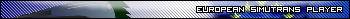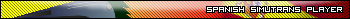## News:

Want to praise Simutrans?
Your feedback is important for us.

## [Ajuda] Vagão Fica no depot como locomotiva

Started by Fox92, December 18, 2009, 11:27:46 PM

0 Members and 1 Guest are viewing this topic.

#### Fox92

Estava criando um vagão para combustivel, mas ele aparece como locomotiva:

`obj=vehiclename=VagãoCombustivelcopyright=Fox92intro_month=1intro_year=2400runningcost=150cost=2000000weight=4gear=120power=30speed=300payload=55freight=Oellength=9waytype=trackengine_type=electric`

se alguem puder me orientar.
Visitem esse Blog-----> http://www.falandocomasparedes.co.cc/

#### vilvoh

#1
Os vagãos não deve ter nenhum desses atributos no arquivo dat:

• engine_type
• gear
• power

Alguns exemplos de vagãos criados com os atributos corretos:

`obj=vehiclename=OldOillength=6copyright=uzurpatorwaytype=trackfreight=Oelpayload=18speed=90cost=70000weight=9RunningCost=200intro_year=1865########################obj=vehiclename=Oelwagenwaytype=trackfreight=Oelcopyright=DanMacKpayload=45cost=73000weight=18speed=120RunningCost=850sound=-1intro_year=1939############################obj=vehiclename=NeuOelwagenwaytype=trackfreight=Oelpayload=80cost=134000weight=23speed=100RunningCost=850sound=-1intro_year=1951`Escala Real...a blog about Simutrans in Spanish...#2